Thermodynamics and Propulsion
Subsections

6.9 Effect of Departures from Ideal Behavior -- Real Cycle behavior

To conclude this chapter, we will now improve our estimates of cycle performance by including the effects of irreversibility. We will use the Brayton cycle as an example. What are the sources of non-ideal performance and departures from reversibility?

• Losses (entropy production) in the compressor and the turbine.
• Stagnation pressure decrease in the combustor.
• Heat transfer.

We take into account here only irreversibility in the compressor and in the turbine. Because of these irreversibilities, we need more work,(the changes in kinetic energy from inlet to exit of the compressor are neglected), to drive the compressor than in the ideal situation. We also get less work,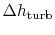, back from the turbine. The consequence, as can be inferred from Figure 6.16, is that the net work from the engine is less than in the cycle with ideal components.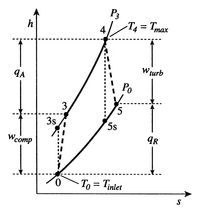To develop a quantitative description of the effect of these departures from reversible behavior, consider a perfect gas with constant specific heats and neglect kinetic energy at the inlet and exit of the turbine and compressor. We define the turbine adiabatic efficiency aswhereis specified to be at the same pressure ratio as. There is a similar metric for the compressor, the compressor adiabatic efficiency: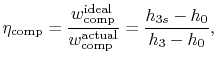again for the same pressure ratio. Note that for the turbine the ratio is the actual work delivered divided by the ideal work, whereas for the compressor the ratio is the ideal work needed divided by the actual work required. These are not thermal efficiencies, but rather measures of the degree to which the compression and expansion approach the ideal processes.

We now wish to find the net work done in the cycle and the efficiency. The net work is given either by the difference between the heat received and rejected or the work of the compressor and turbine, where the convention is that heat received is positive and heat rejected is negative and work done is positive and work absorbed is negative.The thermal efficiency is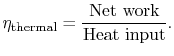We need to calculate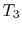,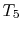.

From the definition of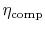,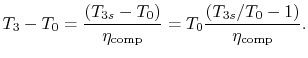WithSimilarly, by the definitionwe can find:The thermal efficiency can now be found:With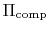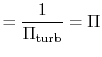and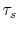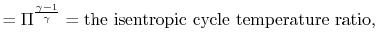orThere are several non-dimensional parameters that appear in this expression for thermal efficiency. We list these in the two sections below and show their effects in accompanying figures.

6.9.1 Parameters reflecting design choices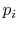: cycle pressure ratio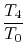6.9.2 Parameters reflecting the ability to design and execute efficient componentsIn addition to efficiency, net rate of work is a quantity we need to examine,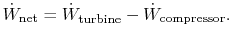Putting this in a non-dimensional form,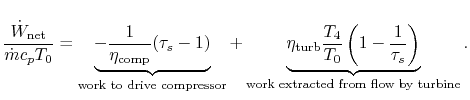[Non-dimensional work as a function of cycle pressure ratio for different values of turbine entry temperature divided by compressor entry temperature]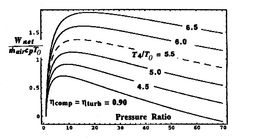[Overall cycle efficiency as a function of pressure ratio for different values of turbine entry temperature divided by compressor entry temperature][Overall cycle efficiency as a function of cycle pressure ratio for different component efficiencies]Trends in net power and efficiency are shown in Figure 6.17 for parameters typical of advanced civil engines. Some points to note in the figure:

• For any,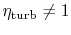the optimum pressure ratiofor maximumis not the highest that can be achieved, as it is for the ideal Brayton cycle. The ideal analysis is too idealized in this regard. The highest efficiency also occurs closer to the pressure ratio for maximum power than in the case of an ideal cycle. Choosing this as a design criterion will therefore not lead to the efficiency penalty inferred from ideal cycle analysis.
• There is a strong sensitivity to the component efficiencies. For example, for, the cycle efficiency is roughly two-thirds of the ideal value.
• The maximum power occurs at a value ofor pressure ratioless than that for max(this trend is captured by ideal analysis).
• The maximum power and maximumare strongly dependent on the maximum temperature,.

A scale diagram of a Brayton cycle with non-ideal compressor and turbine behaviors, in terms of temperature-entropy (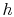-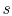) and pressure-volume (-) coordinates is given below as Figure 6.18.

 []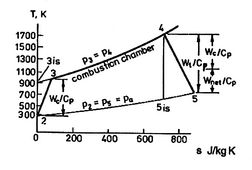[]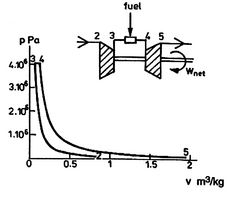Muddy Points

Isn't it possible for the mixing of two gases to go from the final state to the initial state? If you have two gases in a box, they should eventually separate by density, right? (MP 6.22)

How canbe the maximum turbine inlet temperature? (MP 6.23)

When there are losses in the turbine that shift the expansion in-diagram to the right, does this mean there is more work than ideal since the area is greater? (MP 6.24)

For an afterburning engine, why must the nozzle throat area increase if the temperature of the fluid is increased? (MP 6.25)

Why doesn't the pressure in the afterburner go up if heat is added? (MP 6.26)

Why is the flow in the nozzle choked? (MP 6.27)

What's the point of having a throat if it creates a retarding force? (MP 6.28)

Why isn't the stagnation temperature conserved in this steady flow? (MP 6.29)

Douglas Quattrochi 2006-08-06